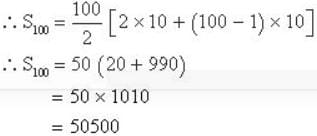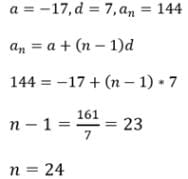# Test: Arithmetic Progressions

## 10 Questions MCQ Test Mathematics For JEE | Test: Arithmetic Progressions

Description
Attempt Test: Arithmetic Progressions | 10 questions in 10 minutes | Mock test for JEE preparation | Free important questions MCQ to study Mathematics For JEE for JEE Exam | Download free PDF with solutions
QUESTION: 1

Solution:

By formula
Sn = n/2(a+l)

QUESTION: 2

### Find the sum of first hundred even natural numbers which are divisible by 5.

Solution:

Even natural numbers which are divisible by 5 are 10,20.30...
Difference between the consecutive terms is same
∴ They form an AP whose first term (a) = 10,
common on difference (d)  = 10,
and number of terms (n) = 100∴ The sum of first hundred even natural numbers which are divisible by 5  is 50500.

QUESTION: 3

### If the 10 times of the 10th term of an AP is equal to 15 times to the 15th term, then the 25th term is:

Solution:

10 x 10th term = 15 x 15th term
let a is the first term and d is the common difference .
10(a+9d) = 15(a+14d)
5a + 120d = 0
a + 24d = 0
now , 25th term = a + (25-1)d
= a+24d = 0
hence 25th term = 0

QUESTION: 4

Find the missing number. 1, 4, 9, 16, 25, 36, 49, (....)

Solution:

The series is 12, 22, 32, 42, 52, 62, 72, ...
Hence, next term = 82 = 64

QUESTION: 5

The first negative term of the  A.P.62,57,52…. is the

Solution:

a = 62  d = 57 - 62 = -5
tn = a + (n-1)d
= 62 + (n-1)(-5)
= 62 - 5n + 5
= 67 - 5n
From the options, we take '14'
= 67 - 5(14)
= 67 - 70
= -3 (The first negative term will be at the 14th term)

QUESTION: 6

How many terms of the series 24,20,16,…are required so that their sum is 72?

Solution:

The series is 24,20,16...in AP
so a = 1st term=24 & common difference = 20-24 = -4
sum = 72 is of say n terms
then 72 = n/2[2*24+(n-1)*-4] = n/2[48-4n+4] = n/2[52-4n]
or 144 = 52n-4n2

or 4n2-52n+144 = 0
or n2-13n+36 = 0
or n2-9n-4n+36 = 0
or n(n-9)-4(n-9) = 0
or (n-9)(n-4) = 0
or n = 9 or 4

QUESTION: 7

Roots of quadratic equation x2 – 3x = 0 , will be

Solution:

Given x 2 - 3x = 0
Factor x out in the expression on the left.
x (x - 3) = 0
For the product x (x - 3) to be equal to zero we nedd to have
x = 0 or x - 3 = 0
Solve the above simple equations to obtain the solutions.
x = 0
or
x = 3

QUESTION: 8

The number of terms in the sequence -17, -10, -3,…., 144 is:

Solution:QUESTION: 9

Three terms in A.P. are such that their sum is 45. What is the middle term?

Solution:

Let the three numbers be a-d, a, a+d
ATQ,
a - d + a + a + 2d = 45
3a = 45
a = 15
Middle term is 15

QUESTION: 10

The terms of an A.P. are doubled, then the resulting sequence is

Solution:

The general form of an AP is  a,  a+d, a+2d,.....
where a is the first term and d is the common difference
If we double the terms,  the new sequence would be
A , a+2d, a+4d,......
We can observe that this sequence is also an AP
First term is a
Common difference is 2d  nth term= 2a+(n-1)2d
= 2[a+(n-1)d]Use Code STAYHOME200 and get INR 200 additional OFF Use Coupon Code